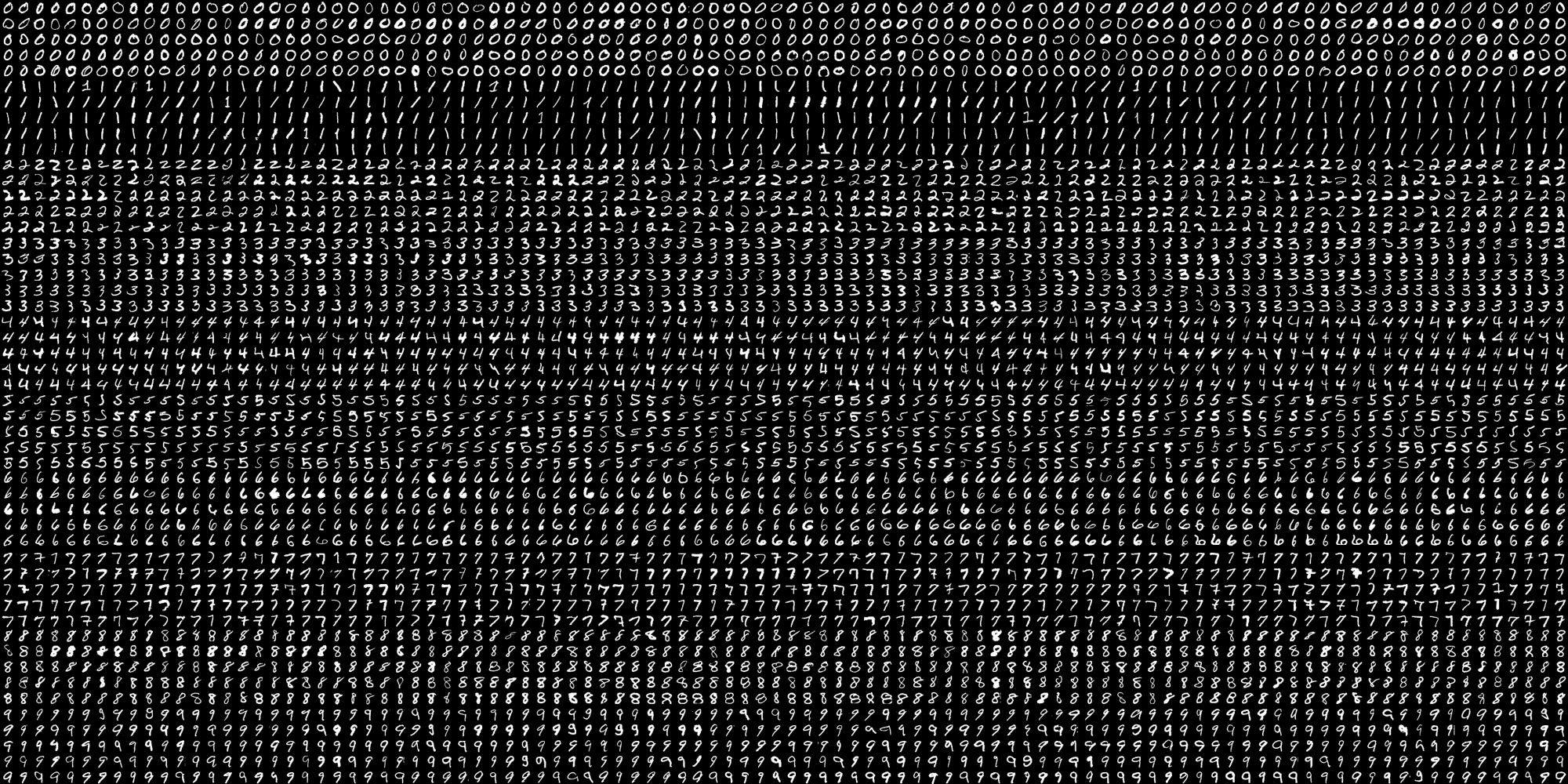# OCR of Handwritten digits | OpenCV

OCR which stands for Optical Character Recognition is a computer vision technique used to identify the different types of handwritten digits that are used in common mathematics. To perform OCR in OpenCV we will use the KNN algorithm which detects the nearest k neighbors of a particular data point and then classifies that data point based on the class type detected for n neighbors.

Data UsedThis data contains 5000 handwritten digits where there are 500 digits for every type of digit. Each digit is of 20×20 pixel dimensions. We will split the data such that 250 digits are for training and 250 digits are for testing for every class.

Below is the implementation.

 `import` `numpy as np ` `import` `cv2 ` `  `  `     `  `# Read the image ` `image ``=` `cv2.imread(``'digits.png'``) ` ` `  `# gray scale conversion ` `gray_img ``=` `cv2.cvtColor(image, ` `                        ``cv2.COLOR_BGR2GRAY) ` ` `  `# We will divide the image ` `# into 5000 small dimensions  ` `# of size 20x20 ` `divisions ``=` `list``(np.hsplit(i,``100``) ``for` `i ``in` `np.vsplit(gray_img,``50``)) ` ` `  `# Convert into Numpy array ` `# of size (50,100,20,20) ` `NP_array ``=` `np.array(divisions) ` `  `  `# Preparing train_data ` `# and test_data. ` `# Size will be (2500,20x20) ` `train_data ``=` `NP_array[:,:``50``].reshape(``-``1``,``400``).astype(np.float32) ` ` `  `# Size will be (2500,20x20) ` `test_data ``=` `NP_array[:,``50``:``100``].reshape(``-``1``,``400``).astype(np.float32) ` ` `  `# Create 10 different labels  ` `# for each type of digit ` `k ``=` `np.arange(``10``) ` `train_labels ``=` `np.repeat(k,``250``)[:,np.newaxis] ` `test_labels ``=` `np.repeat(k,``250``)[:,np.newaxis] ` `  `  `# Initiate kNN classifier ` `knn ``=` `cv2.ml.KNearest_create() ` ` `  `# perform training of data ` `knn.train(train_data, ` `          ``cv2.ml.ROW_SAMPLE,  ` `          ``train_labels) ` `  `  `# obtain the output from the ` `# classifier by specifying the ` `# number of neighbors. ` `ret, output ,neighbours, ` `distance ``=` `knn.findNearest(test_data, k ``=` `3``) ` `  `  `# Check the performance and ` `# accuracy of the classifier. ` `# Compare the output with test_labels ` `# to find out how many are wrong. ` `matched ``=` `output``=``=``test_labels ` `correct_OP ``=` `np.count_nonzero(matched) ` `  `  `#Calculate the accuracy. ` `accuracy ``=` `(correct_OP``*``100.0``)``/``(output.size) ` `  `  `# Display accuracy. ` `print``(accuracy) `

Output

`91.64`

Attention geek! Strengthen your foundations with the Python Programming Foundation Course and learn the basics.

To begin with, your interview preparations Enhance your Data Structures concepts with the Python DS Course.

My Personal Notes arrow_drop_upCheck out this Author's contributed articles.

If you like GeeksforGeeks and would like to contribute, you can also write an article using contribute.geeksforgeeks.org or mail your article to contribute@geeksforgeeks.org. See your article appearing on the GeeksforGeeks main page and help other Geeks.

Please Improve this article if you find anything incorrect by clicking on the "Improve Article" button below.

Article Tags :

Be the First to upvote.

Please write to us at contribute@geeksforgeeks.org to report any issue with the above content.